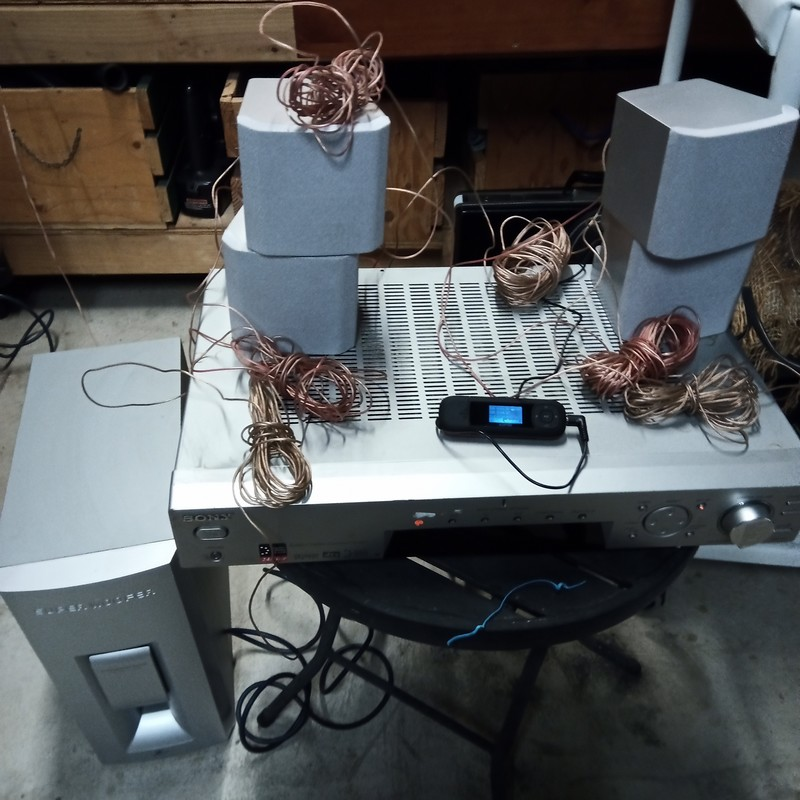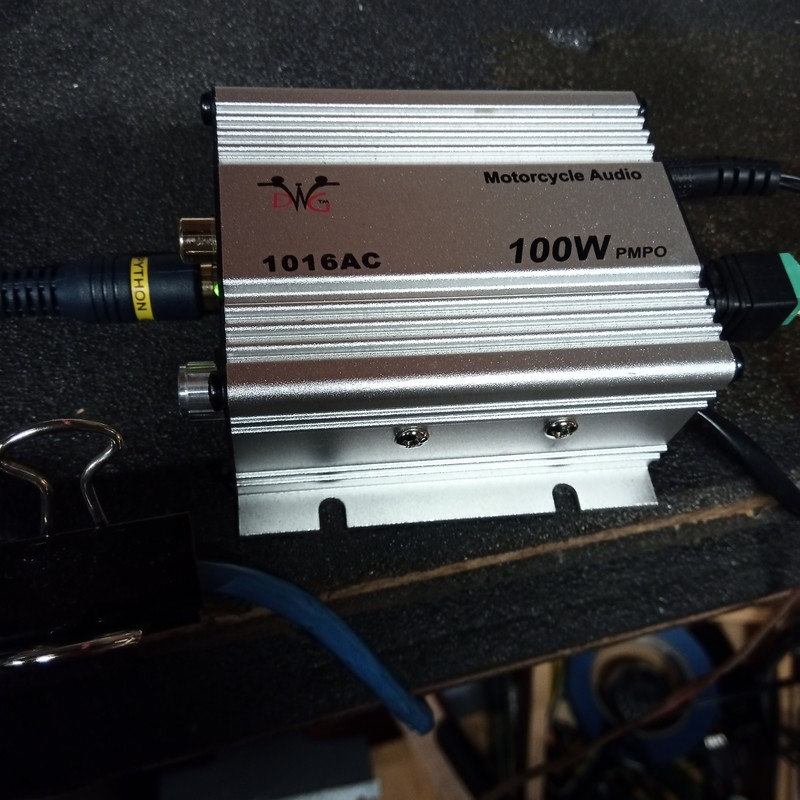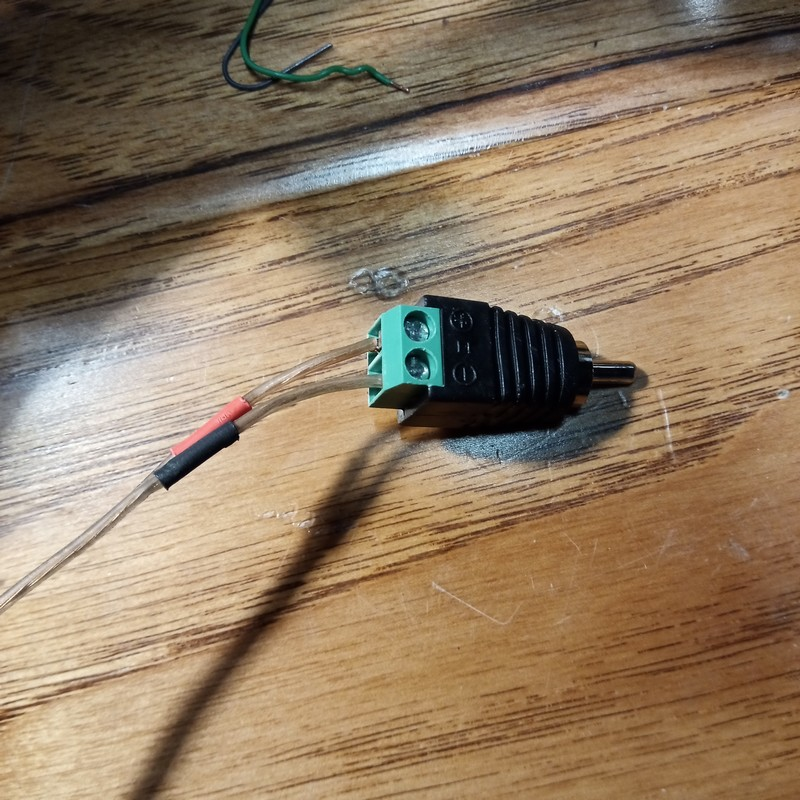```
[=x=-=x=-=x=-=x=-=x=-=x=-=x=-=x=-=x=-=x=-=x=-=x=-=x=-=x=-=x=-=x=-=x=]
[<<>><<>><<>><<>><<>><<>><<>><<>><<>><<>><<>><<>><<>><<>><<>><<>><<>]
[=x=-=x=-=x=-=x=-=x=-=x=-=x=-=x=-=x=-=x=-=x=-=x=-=x=-=x=-=x=-=x=-=x=]

Stardate: 20210323.2039
Location: xiled rumintation concentrator
Input Device: Repurposed Lenovo ThinkCentre
Visual: concentrator interior
Emotional:  fed, groggy

[=x=-=x=-=x=-=x=-=x=-=x=-=x=-=x=-=x=-=x=-=x=-=x=-=x=-=x=-=x=-=x=-=x=]

Learning myself a little about speakers.  Currently, I am testing
audio in the xiled rumination concentrator.  So far, it sounds
pretty good.  There is a salvaged Sony receiver (Model STR-SL7)
that I picked up from the dumpster at work.  It works, but the
digital display does not so I have to fuss with the menus blind.
Good thing I found the manual online.  It also has a big dent on the
top.

There is a set of Zenith home theatre speakers and subwoofer attached
to it that were left behind by the previous owner of my house.  The
receiver requires speakers with a range of 8-16 ohms, but the
speakers are only 4 ohms.  I ended up wiring the speakers in a series
(for a total of 8ohms) and only having the left and right channels
+ subwoofer, with 2 speakers facing me and 2 speakers + subwoofer
behind me in the concentrator.  The wiring is basically this
(sorry for lame drawing...need Visio for gopher):

___________
[ SONY     ]
[          ]    [Left Speaker (rear)]      [Left Speaker (front)]
[Left POS  ]->->[POS]->->4ohms->[NEG]->->->[POS]->-4ohms->->[NEG]->->
[          ]                                                        /
[Left NEG  ]<-<-<-<-<-<-<-<-<-<-<-<-<-<-<-<-<-<-<-8ohms<-<-<-<-<-<-<
[          ]
[          ]
[Right POS ]->->[POS]->->4ohms->-[NEG]->->-[POS]->-4ohms->->-[NEG]->->
[          ]    [Right Speaker (rear)]     [Right Speaker (front)]  /
[          ]                                                       /
[Right NEG ]<-<-<-<-<-<-<-<-<-<-<-<-<-<-<-<-<-<-<-8ohms<-<-<-<-<-<-
[          ]
[SUBWOOFER ]
[  OUT     ]->->->->->[Subwoofer Amp]->->->[Passive Subwoofer]
[__________]

->-> <-<- is flow of power

The Zenith subwoofer is passive but the receiver requires an active
subwoofer.   I have an old amp that I salvaged after I totaled my
bike a few years ago, so I hooked that up and it seems to work
perfectly.  Not sure if it's bad to use that, but that's what I've
got.

Yeah, the setup is a bit much, but I got to learn stuff and now have
nice sound and thumpin' bass from parts that would have otherwise
ended up in the landfill.

Here's some pics: